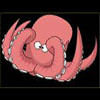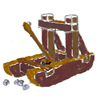#### You may also like### Base Puzzle

This investigation is about happy numbers in the World of the Octopus where all numbers are written in base 8 .Octi the octopus counts.### Basically

The number 3723(in base 10) is written as 123 in another base. What is that base?### Oh for the Mathematics of Yesteryear

A garrison of 600 men has just enough bread ... but, with the news that the enemy was planning an attack... How many ounces of bread a day must each man in the garrison be allowed, to hold out 45 days against the siege of the enemy?

# Happy Octopus

##### Age 11 to 14Challenge Level

This is an investigation of sequences formed by writing down the sum of the squares of the digits of each number to get the next number in the sequence. A number is called happy if it starts a sequence that goes to a number which is repeated over and over again (e.g. 1, 1, 1, 1, $\ldots$) and this is called a fixed point. Other numbers lead to cyclic patterns which repeat over and over again. For example this sequence leads to a repeating 8-cycle:

25, 29, 85, 89, 145, 42, 20, 4, 16, 37, 58, 89, $\ldots$

Rachel Walker from the Mount School, York explained that in base ten, 122 is a sad number because it has an 8-cycle like 25 and this is her solution:

122, 9, 81, 65, 37, 58, 89, 145, 42, 20, 4, 16, 37, 58........

You can find a lot of 'happy numbers' such as: 310, 70, 86, 130, 7, 5555, 1212, 13, 1000 and other numbers which are variations of these, e.g. swapping the numbers the other way around (13, 31) and adding zeros onto the end of numbers (31, 310). Also you get numbers that add up to other happy numbers e.g. 5555 - four fives squared = 100.

The following sequences are the patterns of fixed points and cycles in base eight that I have found. These are fixed points so they are happy numbers and any numbers which start sequences ending like this are happy numbers.
64, 64, 62, 64, 64, $\ldots$
24, 24, 24, 24, 24, $\ldots$
1, 1, 1, 1, ,1, $\ldots$

These are 2-cycles:
32, 15, 32, 15, 32, 15, $\ldots$
20, 4, 20, 4, 20, 4, $\ldots$

This is a 3-cycle:
31, 12, 5, 31, 12, 5, 31, 12, 5, $\ldots$

Pen Areecharoenlert, also from The Mount School, York found some more happy numbers and cycles in base eight. Pen says "There does not appear to be any pattern in which ones are happy, but it looks as though there are 3 fixed points: 1, 24 and 64." This is Pen's list.

1, 1, 1, $\ldots$ so 1 is happy.
2, 4, 20, 4, 20, $\ldots$ so 2 is sad. (2-cycle)
3, 11, 2, 4, 20, $\ldots$ so 3 is sad. (2-cycle)
5, 31, 12, 5, $\ldots$ so 5 is sad. (3-cycle)
6, 44, 40, 20, 4, 20, $\ldots$ so 6 is sad. (2-cycle)
7, 61, 45, 51, 32, 15, 32, $\ldots$ so 7 is sad. (2-cycle)
10, 1, 1, 1, 1$\ldots$ so 10 is happy.
11, 2, 4, 20, 4, $\ldots$ so 11 is sad. (2-cycle)
12, 5, 31, 12, $\ldots$ so 12 is sad. (3-cycle)
13, 12, $\ldots$ so 13 is sad. (3-cycle)
14, 21, 5, $\ldots$ so 14 is sad. (3-cycle)
5, 32, 15,$\ldots$ so 15 is sad. (2-cycle)
16, 45, 51, 32, $\ldots$ so 16 is sad. (2-cycle)
17, 62, 50, 31,$\ldots$ so 17 is sad. (3-cycle)
20, 4, 20, 4, $\ldots$ so 20 is sad. (2-cycle)
21, 5, $\ldots$ so 21 is sad. (3-cycle)
22, 10, 1, 1,$\ldots$ so 22 is happy.
23, 15, $\ldots$ so 23 is sad. (2-cycle)
24, 24, 24, 24, so 24 is happy.
25, 35,, 42, 24, 24,$\ldots$ so 25 is happy.
26, 50, 31,$\ldots$ so 26 is sad. (3-cycle)
27, 65, 75, 112, 6, 44, 40, 20,$\ldots$ so 27 is sad. (2-cycle)
30 , 11, 2, 4, 20, $\ldots$ so 30 is sad. (2-cycle)
31, 12, $\ldots$ so 31 is sad. (3-cycle)
32, 15, $\ldots$ so 32 is sad. (2-cycle)
33, 22, 10, 1, $\ldots$ so 33 is happy.
34, 31, 12, $\ldots$ so 34 is sad. (3-cycle)
35, 42, 24, 24, $\ldots$ so 35 is happy.
36, 55, 62, 50, 31, $\ldots$ so 36 is sad. (3-cycle)
37, 72, 65, 75, 112 so 37 is sad. (2-cycle)
40 > 20 > 4 > 20 so 40 is sad. . (2-cycle)
41, 21, 5, 31, 12, 5, $\ldots$ so 41 is sad. (3-cycle)
42, 24, 24,$\ldots$ so 42 is happy.
43, 31, $\ldots$ so 43 is sad. (3-cycle)
44, 40, 20, 4, 20,$\ldots$ so 44 is sad. (2-cycle)
45, 51, 32, $\ldots$ so 45 is sad. (2-cycle)
46, 64, 64, 64, $\ldots$ so 46 is happy.
47, 101, 2, 4, 20, $\ldots$ so 47 is sad. (2-cycle)

Claire Kruithof, Madras College, St Andrews proved that 24 and 64 are the only 2 digit fixed points in base 8. This is Claire's proof:

"We wanted to see if there would be any more fixed points so we used algebra. The number following $ab$ is $a^2 + b^2$ where $a$ and $b$ are between 0 and 7. For a fixed point we have:

$a^2 + b^2 = 8a + b$ $b^2 - b = 8a - a^2$ $b(b - 1) = a (8 - a)$

Searching for possibilities
 B a 1 x 0 = 0 7 x 1 = 7 2 x 1 = 2 6 x 2 = 12 3 x 2 = 6 5 x 3 = 15 4 x 3 = 12 4 x 4 = 16 5 x 4 = 20 6 x 5 = 30 7 x 6 = 42

The only possibilities are $a = 2, \ b = 4$ and $a = 6, \ b = 4$.

With more digits the sums of squares are never bigger than some multiple of $7^2$. It can be proved that sequences cannot go on for ever without repeating patterns and so all sequences go into cycles or go to a fixed point. Moreover it can be proved that there are no fixed points with 3 or more digits.